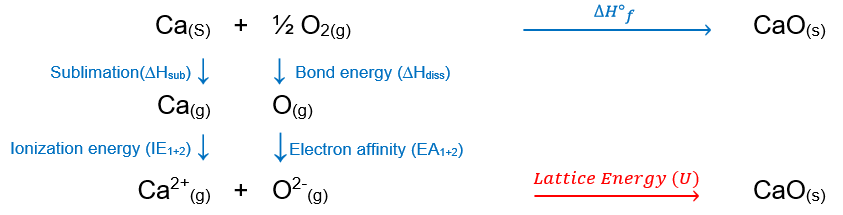# Problem: Use the Born-Haber cycle to calculate the lattice energy of CaO. You may need the following data: electron affinity of oxygen EA1 = −141 kJ/mol, EA2 = 744 kJ/mol ionization energy of calcium IE1 = 590 kJ/mol, IE2 = 1145 kJ/mol the enthalpy of sublimation for calcium ΔHsub = 178kJ/molbond energy of O2 ΔHdiss = 498 kJ/molM(s) + ½ X2(g) → MX(s), ΔH°f = −635.09 kJ/mol

###### FREE Expert Solution

Recall that lattice energy is the energy required to combine two gaseous ions into a solid ionic compound:

Ca2+(g) + O2(g)  CaO(s)

To calculate for lattice energy, we need to do the Born-Haber cycle for CaO

Ca(s) + ½ O2(g)  CaO(s), ΔH˚f82% (53 ratings)###### Problem Details

Use the Born-Haber cycle to calculate the lattice energy of CaO. You may need the following data:

electron affinity of oxygen EA1 = −141 kJ/mol, EA2 = 744 kJ/mol

ionization energy of calcium IE1 = 590 kJ/mol, IE2 = 1145 kJ/mol

the enthalpy of sublimation for calcium ΔHsub = 178kJ/mol

bond energy of O2 ΔHdiss = 498 kJ/mol

M(s) + ½ X2(g) → MX(s), ΔH°f = −635.09 kJ/mol